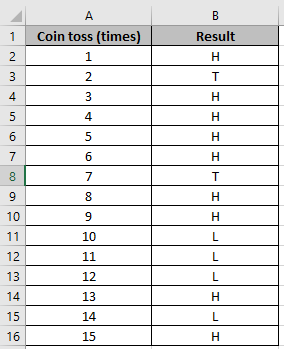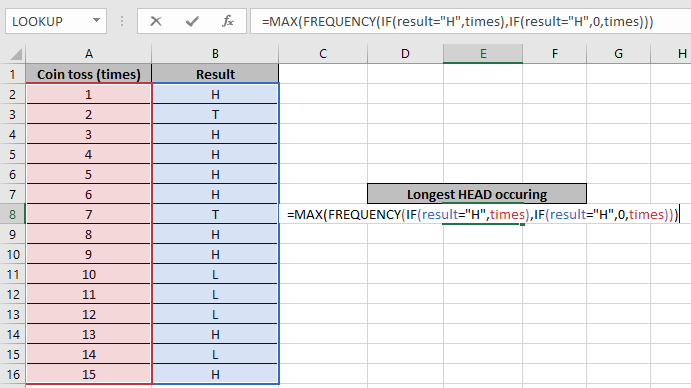# Longest Toss winning streak

In this article, we will learn how to get the Longest Toss winning streak in Excel.

For instance, you have a large data of a team of a particular season's wins or losses. Or getting to know how many consecutive times HEADS occur in the coin toss session. Get the formula to calculate the longest winning streak of the session.

For this article we will be needing the use of the following functions:

Generic formula for getting the longest winning streak:

 = {MAX ( FREQUENCY ( IF ( result = "H" , times ) , IF ( result = "H" , 0 , times ) ) )}

Explanation:

• IF ( result = "H" , times ) returns an array of the count of wins ID and FALSE values.
• IF ( result = "H" , 0 , times ) returns array of 0s and count of losses ID.
• Now FREQUENCY function returns the frequency distribution of each array element in the second array.
• Finally, MAX function will return the max occurrence of the value.

Note: Curly braces in excel must not be given manually instead use CTRL + SHIFT + ENTER.

Let's jump into an example to make things clear:

Example: Get Longest Streak of Heads in a Toss Using Frequency Distribution

John has a coin and he tosses the coin at times and records their respective results in the adjacent cell. We need to find out what the maximum times consecutive heads occur.Now we will use the below formula to get the MAX count for the data

Formula:

 { = MAX ( FREQUENCY ( IF ( result = "H" , times ) , IF ( result = "H" , 0, times ) ) ) }

Explanation:

• IF ( result = "H" , times ) returns an array of the count of wins ID and FALSE values. {1;FALSE;3;4;5;6;FALSE;8;9;FALSE;FALSE;FALSE;13;FALSE;15}
• IF ( result = "H" , 0 , times ) returns array of 0s and count of losses ID. {0;2;0;0;0;0;7;0;0;10;11;12;0;14;0}
• Now FREQUENCY function returns the frequency of each array element in the second array. =MAX({0;1;0;0;0;0;4;0;0;2;0;0;0;1;0;1})
• MAX function returns the max occurrence of the value.Here result is named range used for the array B2:B16 & times is the named range used for the array A2:A16. Press Ctrl + Shift + Enter to get the result.The function returns #VALUE error if the formula doesn't conclude with Ctrl + Shift + Enter shortcut.

As you can see using the above formula, you can get the max consecutive occurrence. Below are some of the observational results.

Notes:

1. The function considers non- numeric values as 0s.
2. The function considers logic value TRUE as 1 and FALSE as 0.
3. The argument array must be of the same length else the function returns error.
4. The function returns 0 if the  Ctrl + Shift + Enter for curly braces is not used.

Hope this article about how to Longest Toss a winning streak in Excel is explanatory. Find more articles on FREQUENCY functions here. Please share your query below in the comment box. We will assist you.

Related Articles

How to use the FREQUENCY function in Excel

How to use the MAX function in Excel

How to use the IF function in excel

How to Remove Text in Excel Starting From a Position

How to use the ROWS function in Excel

Validation of text entries

Create a drop down list in excel with colour

Remove lead and trailing spaces from text in Excel

Popular Articles

50 Excel Shortcuts to Increase Your Productivity

Edit a dropdown list

Absolute reference in Excel

If with conditional formatting

If with wildcards

Vlookup by date

Convert Inches To Feet and Inches in Excel 2016

Join first and last name in excel

Count cells which match either A or B

Terms and Conditions of use

The applications/code on this site are distributed as is and without warranties or liability. In no event shall the owner of the copyrights, or the authors of the applications/code be liable for any loss of profit, any problems or any damage resulting from the use or evaluation of the applications/code.